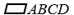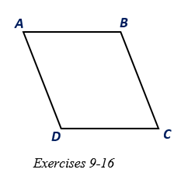Chapter 4.1, Problem 15E### Elementary Geometry for College St...

6th Edition
Daniel C. Alexander + 1 other
ISBN: 9781285195698

#### Solutions

Chapter
Section### Elementary Geometry for College St...

6th Edition
Daniel C. Alexander + 1 other
ISBN: 9781285195698
Textbook Problem
1 views

# Assuming that m ∠ B >   m ∠ A in, which diagonal ( A C -   o r   B D - ) would be longer?To determine

To describe:

The longer diagonal between AC- or BD- in ABCD.

Explanation

Given:

Assuming that mB> mA in ABCD and the diagram as shown below.

Corollary:

1. The opposite angles of a parallelogram are congruent.

2. Two consecutive angles of a parallelogram are supplementary.

Approach:

In the given parallelogram ABCD, given that mB> mA.

As per the corollary that the opposite angles of a parallelogram are congruent, mA=mC

and mB=mD.

Join the diagonals AC- or BD-

### Still sussing out bartleby?

Check out a sample textbook solution.

See a sample solution

#### The Solution to Your Study Problems

Bartleby provides explanations to thousands of textbook problems written by our experts, many with advanced degrees!

Get Started

#### Domain Find the domain of the expression. 11. x+3

Precalculus: Mathematics for Calculus (Standalone Book)

#### Evaluate limx1x10001x1.

Calculus: Early Transcendentals

#### What is the value of (X + 1) for the following scores: 0, 1, 4, 2? a. 8 b. 9 c. 11 d. 16

Essentials of Statistics for The Behavioral Sciences (MindTap Course List)

#### Describe the strengths and weaknesses of physiological measures.

Research Methods for the Behavioral Sciences (MindTap Course List)